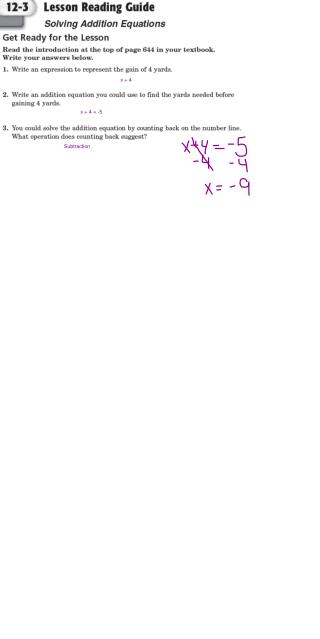DownloadDownload Presentationx + 4

# x + 4

Télécharger la présentation## x + 4

- - - - - - - - - - - - - - - - - - - - - - - - - - - E N D - - - - - - - - - - - - - - - - - - - - - - - - - - -
##### Presentation Transcript

1. x + 4 x + 4 = -5 Subtraction

2. what you do to one side, you must do to the other!

4. what you do to one side, you must do to the other!!

6. Using Models 7 + n = 12 + n =

7. Using Models n - 5 = 9 - = n

8. Using Models + = n

9. Using Models - n =

14. What are the missing values? 15 + 9 = 24 96 + 14 = 110 56 - 35 = 21 82 - 6 = 76 37 - 24 = 13 24 - 12 = 12

15. Solving addition equations x + 5 = 11 x = ? x + 5 = 11 x + 5 - 5 = 11- 5 x x = 6 6 + 5 = 11

16. Model the equation x x Write an equation: Write an equation: = = Solve the equation: Solve the equation: 11 = x + 9 x + 3 = 7 11 - 9 = x + 9 - 9 x + 3 - 3 = 7 - 3 x = 2 x = 4 4 + 3 = 7 11 = 2 + 9

17. Solving subtraction equations x - 9 = 47 x - 51 = 33 x - 51 + 51 = 33 + 51 ? ? x - 9 + 9 = 47 + 9 ? x = 84 x = 56 84 - 51 = 33 56 - 9 = 47

18. 2 2 + v = 4 Pull Pull d + 8 = 13 5 Pull Pull s - 5 = 2 7 Pull Pull 11 + x = 15 4 Pull Pull

19. 3 7 + p = 10 Pull Pull b - 7 = 11 18 Pull Pull l + 3 = 5 2 Pull Pull g - 5 = 6 11 Pull Pull

20. b+5, subtract 5 is the inverse operation subtract 5 from both sides of the equation, the 2 sides remain equal says that the sum of any # & 0 is that #. So, b+0=-3 is b=-3 that you checked your answer Remember What You Learned 8) inverse = opposite

21. b/c of the inverse operation used to get a variable alone y-4, inverse operation is to add 4 to get the variable alone when you add 4 to each side of the equation, the 2 sides remain equal add 4 to each side of the equation